# High School Math : Finding Symmetries

## Example Questions

### Example Question #11 : Pre Calculus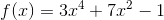This function is:

not symmetric

Explanation:

A function's symmetry is related to its classification as even, odd, or neither.

Even functions obey the following rule: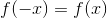Because of this, even functions are symmetric about the y-axis.

Odd functions obey the following rule: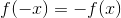Because of this, odd functions are symmetric about the origin.

If a function does not obey either rule, it is neither odd nor even. (A graph that is symmetric about the x-axis is not a function, because it does not pass the vertical line test.)

To test for symmetry, simply substitute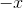into the original equation.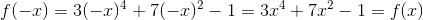Thus, this equation is even and therefore symmetric about the y-axis.